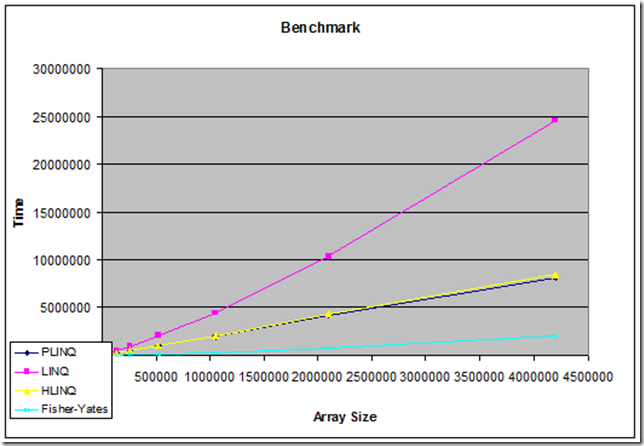# Evolution of Solutions - and Perceived Performance

20/12/2008

I entered a small competition yesterday and wanted to write a short post describing my progress and findings. The competition was rather simple, write a method that returns an array containing the numbers from 1 to 1000 in a random order.

My first solution was the naive LINQ solution.

``````public static int[] NaiveLinq(int max)
{
var random = new Random();
var query = from number in Enumerable.Range(1, max)
orderby random.Next()
select number;
return query.ToArray();
}
``````

I reckoned that several people would post this solution, so I felt like doing something slightly more fancy - and since parallelization is so hot these days, why not do it with PLINQ instead, so it would actually be faster on multi-core systems. The change is really simple.

``````public static int[] PLinq(int max)
{
var random = new Random();
var query = from number in ParallelEnumerable.Range(1, max)
orderby random.Next()
select number;
return query.ToArray();
}
``````

I benchmarked it (on my quad-core machine), and saw that the PLINQ solution was indeed faster - but only for rather big solutions, the overhead was simply too big in small instances of the problem (array size < 3000). This is not too well for a competition that is supposed to make arrays of size 1000. However, reluctant to ditch my parallel idea, I made a hybrid solution, which would use regular LINQ for small instances and PLINQ for big instances, based on my benchmark:

``````public static int[] GenerateUsingHybridLinq(int arrayLength)
{
var random = new Random();
// Use PLINQ if we're above the "very scientific" limit of 3000.
if (arrayLength >= 3000)
{
return (from i in ParallelEnumerable.Range(1, arrayLength)
orderby random.Next()
select i).ToArray();
}
return (from i in Enumerable.Range(1, arrayLength)
orderby random.Next()
select i).ToArray();
}
``````

I posted this solution to the competition and decided I had spent enough time on it. However, it bugged me because I knew that the `Random` class in the .NET framework is not guaranteed to be thread-safe. It also bugged be that I was actually solving a shuffling problem by sorting. Shuffling can be solved in O(n) while sorting is O(n*log(n)). In addition I thought that the LINQ solutions were kind of dull.

So I decided to take a stab at solving it without resolving to sorting. Shuffling is a well known problem, so I implemented the Fisher-Yates algorithm, often used for shuffling cards. It is actually a rather elegant algorithm.

``````public static int[] Generate()
{
Random random = new Random();
var numbers = new int;
// Add sorted numbers to shuffle
for (var i = 0; i < 1000; i++)
numbers[i] = i + 1;
var last = numbers.Length;
while (last > 1)
{
// Select a random entry in the array to swap
var swap = random.Next(last);
// Decrease relevant end of array
last = last - 1;
// Swap numbers using XOR swap, we don't need no stinkin' temp variables
if (last != swap)
{
numbers[last] ^= numbers[swap];
numbers[swap] ^= numbers[last];
numbers[last] ^= numbers[swap];
}
}
return numbers;
}
``````

I adjusted it a bit and finally found a place to make an ode to the awesomeness that is XOR swap, swapping two values without using a temporary variable. Even though the algorithm was faster asymptotically, I was curious how it would venture against the sort-based LINQ solutions performance-wise. Here is the result:Note that both HLINQ and PLINQ use all 4 cores on my quad-core machine. I realize there's an overhead using LINQ, but I'm still impressed how much faster this simple little algorithm is.

I submitted my new solution, with a note to replace my hybrid solution, just before midnight, but unfortunately I think it might have been too late, it didn't seem to make the cut for the competition. At least my hybrid solution made it into the final round - and non-thread-safe Random surely won't be a problem for instances of size 1000 - since it will use regular LINQ for that.